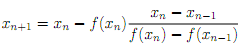## Iterative root fi nding method, MATLAB Programming

Assignment Help:

The secant method is an iterative root nding method that is super-linear. The method has the advantage that it is faster than linear methods and does not require knowledge of the derivative of the function whose root is desired. On the other hand, it requires two starting values. The secant method is de ned by the recurrence relation:Write a function root = secant(f,x1,x2,tol) that computes a root of the function with handle f using the starting values x1 and x2 to within the tolerance tol. The output root must satisfy abs(f(root))

Note: Your roots may di er slightly from those in the test cases, but should satisfy the speci ed tolerance. You are not allowed to use any of Matlab's builtin root nding functions.

#### Statistics, Create a custom chi-square function in matlab, MATLAB in Statis...

Create a custom chi-square function in matlab, MATLAB in Statisticsn..

#### IMAGE PROCESSING, IMAGE PROCESSING TECHNIQUES TO FIND THE HUMAN BLOOD GROUP...

IMAGE PROCESSING TECHNIQUES TO FIND THE HUMAN BLOOD GROUP

#### Minimum cost problem - matlab linear optimization, Minimum Cost Pizza Probl...

Minimum Cost Pizza Problem. Using only the items given in the tables below, create a minimum cost pizza which satis?es both the nutritional requirements of Table 1 and bounds on it

#### Image forgery detection , i have a problem in doing my project which detect...

i have a problem in doing my project which detects image forgery detection using resampling technique any one please help in writing my program

#### Mod function, how to reverse the digits of positive number by using mod fun...

how to reverse the digits of positive number by using mod function?

#### Format function and ellipsis, The Format Function and ellipsis: The de...

The Format Function and ellipsis: The default in MATLAB is to show numbers which have decimal places with four decimal places. The format command can be used to identify the o

#### Draw a calculated y vector on the graph, Create a GUI that has A button to ...

Create a GUI that has A button to bring up a dialog to select a text file and read it in. Plot the x,y values on the GUI Have text entry values, one for each parameter, in which

#### Solution is y., 5y-10+y+56=8y+50+4y

5y-10+y+56=8y+50+4y

#### Implement a matlab function to calculate value, Implement a MatLab function...

Implement a MatLab function to calculate y.  The function should take two input arguments 1) A vector of parameters In the above example this would be of length 3, the first

#### How to create a cell array, how to create a cell array

how to create a cell array